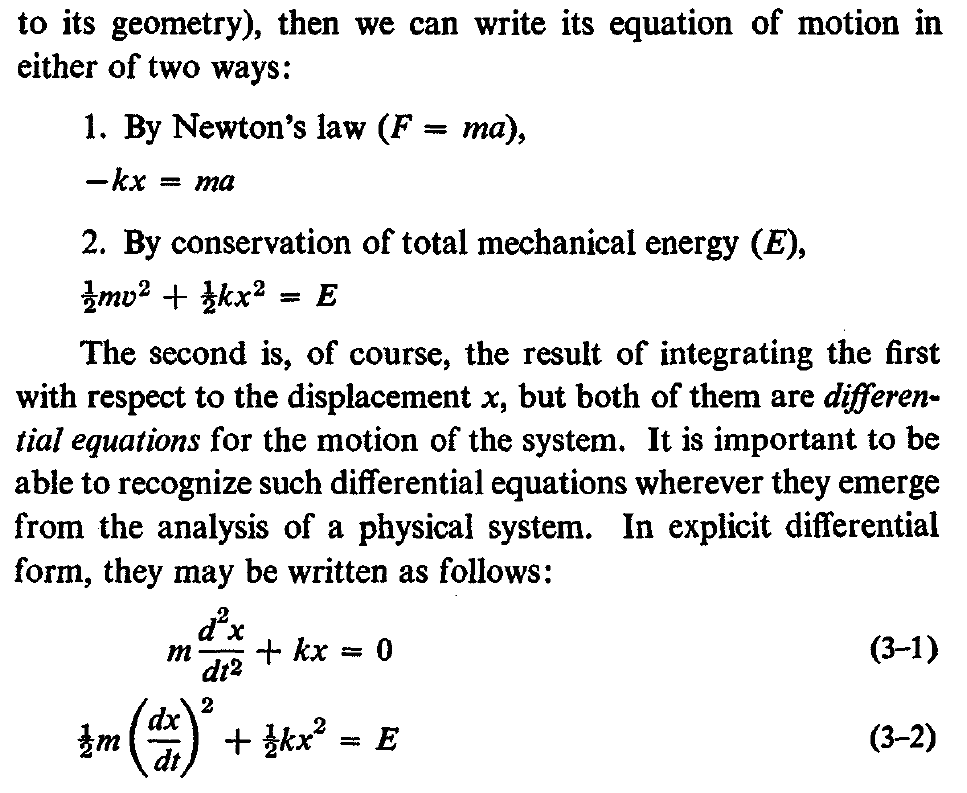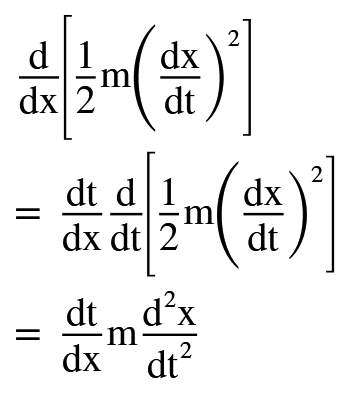# How to integrate d2x/dt2 with respect to x?

• I
• Max Loo Pin Mok
Max Loo Pin Mok
TL;DR Summary
Can we derive one equation of motion from another?
In chapter 3 of Vibrations and Waves by French, there is a description about the equations of motion of a mass-spring system. It was written as shown in the attached picture:Here, m is the mass on the spring, k is the spring constant, x is the extension of the spring, and t is time. My problem is about figuring out how equation (3-1) becomes equation (3-2)? I tried to differentiate 1/2 m(dx/dt)2:I got an extra dt/dx which is not there in equation (3-1). Is my working wrong or is there another way of understanding what is in the textbook: "The second is, of course, the result of integrating the first with respect to the displacement x ..."?

Homework Helper
Gold Member
You are not calculating correctly the derivative $$\frac{d}{dt}\frac{1}{2}m(\frac{dx}{dt})^2$$

if ##f## is a function of ##t##, what is the first derivative of ##f^2##? (w.r.t to ##t##). Then apply this for ##f=\frac{dx}{dt}##

Last edited:
Staff Emeritus
$$a = \frac{dv}{dt} = \frac{dv}{dx} \frac{dx}{dt} = v \frac{dv}{dx},$$ where ##v = dx/dt##.